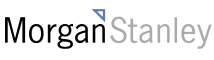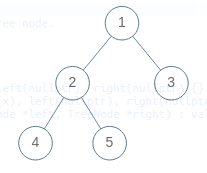New update is available. Click here to update.

# Height of the Binary Tree From Inorder and Level Order Traversal

Medium0/80
10 mins
90 %104 upvotes+4 more

## Problem Statement

#### The height of the binary tree is the number of edges in the longest path from the root node to any leaf node in the tree. In case the tree has only one node, the height is taken to be 0.

Detailed explanation ( Input/output format, Notes, Images )
##### Constraints:
``````1 <= T <= 100
1 <= N <= 3000
1 <= inorder[i] <= N
1 <= levelOrder[i] <= N

Time Limit: 1 sec
``````
##### Sample Input 1:
``````1
5
4 2 5 1 3
1 2 3 4 5
``````
##### Sample Output 1:
``````2
``````
##### Explanation for Sample 1:
``````The binary tree(rooted at node 1) represented by the above inorder and level order traversals is-
````````````We can see that the height of the above binary tree is 2.
``````
##### Sample Input 2:
``````1
7
7 4 2 1 5 3 6
1 2 3 4 5 6 7
``````
##### Sample Output 2:
``````3
``````AutocompleteConsole